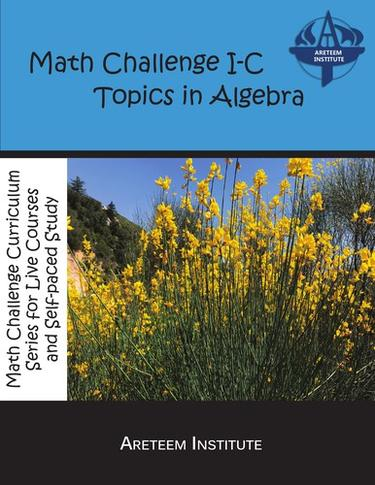# Math Challenge I-C Topics in Algebra

Online textbook for the live course or self-paced study

Areteem Institute
ISBN-13: 9781944863173## eBook Features

or
Rent or Buy from \$ 10.00 USD
Note: We do not guarantee supplemental material with textbooks (e.g. CD's, Music, DVD's, Access Code, or Lab Manuals)

The math challenge curriculum textbook series is designed to help students learn the fundamental mathematical concepts and practice their in-depth problem solving skills with selected exercise problems. Ideally, these textbooks are used together with Areteem Institute's corresponding courses, either taken as live classes or as self-paced classes. According to the experience levels of the students in mathematics, the following courses are offered:

Fun Math Problem Solving for Elementary School (grades 3-5)
Math Challenge I-A Series (grades 6-8; intro to problem solving)
Math Challenge I-B Series (grades 6-8; intro to math contests e.g. AMC 8, ZIML Div M)
Math Challenge I-C Series (grades 6-8; topics bridging middle and high schools)
Math Challenge II-A Series (grades 9+ or younger students preparing for AMC 10)
Math Challenge II-B Series (grades 9+ or younger students preparing for AMC 12)
Math Challenge III Series (preparing for AIME, ZIML Varsity, or equivalent contests)
Math Challenge IV Series (Math Olympiad level problem solving)

These courses are designed and developed by educational experts and industry professionals to bring real world applications into the STEM education. These programs are ideal for students who wish to win in Math Competitions (AMC, AIME, USAMO, IMO, ARML, MathCounts, Math League, Math Olympiad, ZIML, etc.), Science Fairs (County Science Fairs, State Science Fairs, national programs like Intel Science and Engineering Fair, etc.) and Science Olympiad, or purely want to enrich their academic lives by taking more challenges and developing outstanding analytical, logical thinking and creative problem solving skills.

Math Challenge I-C is a four-part course designed to bridge the middle school and high school math materials. For students who participate in the American Math Competitions (AMC), there is a big gap in both the fundamental math concepts and the problem-solving techniques involved between the AMC 8 and AMC 10 contests. This course is developed to help students transition smoothly from middle school to high school, and prepare them for high school math competitions including the AMC 10 \ and 12, ARML, and ZIML. The full course covers topics and introductory problem solving in algebra, geometry, and finite math. Algebraic topics include linear equations, systems of equations and inequalities, exponents and radicals, factoring polynomials, and solving quadratic equations. Geometric topics include angles in triangles, quadrilaterals, and polygons, congruent and similar polygons, calculating area, and algebraic geometry. Topics in finite math include logic, introductory number theory, and an introduction to probability and statistics. These topics serve as the fundamental knowledge needed for a more advanced problem solving course such as Math Challenge II-A.

The course is divided into four terms:

Summer, covering Algebra
Fall, covering covering additional topics in Algebra
Winter, covering Geometry
Spring, covering Finite Math

The book contains course materials for Math Challenge I-C: Additional topics in Algebra.

We recommend that students take all four terms starting with the Summer, but students with the required background are welcome to join for later terms in the course, or select suitable terms for self-paced study.

Students can sign up for the course at https://classes.areteem.org for the live online version or at https://www.edurila.com for the self-paced version.

Sold By Areteem Institute 978-1-944863-17-3, 9781944863173, 1944863176 2018 English 161 Math Challenge I-C Topics in Algebra Areteem Institute www.areteem.org